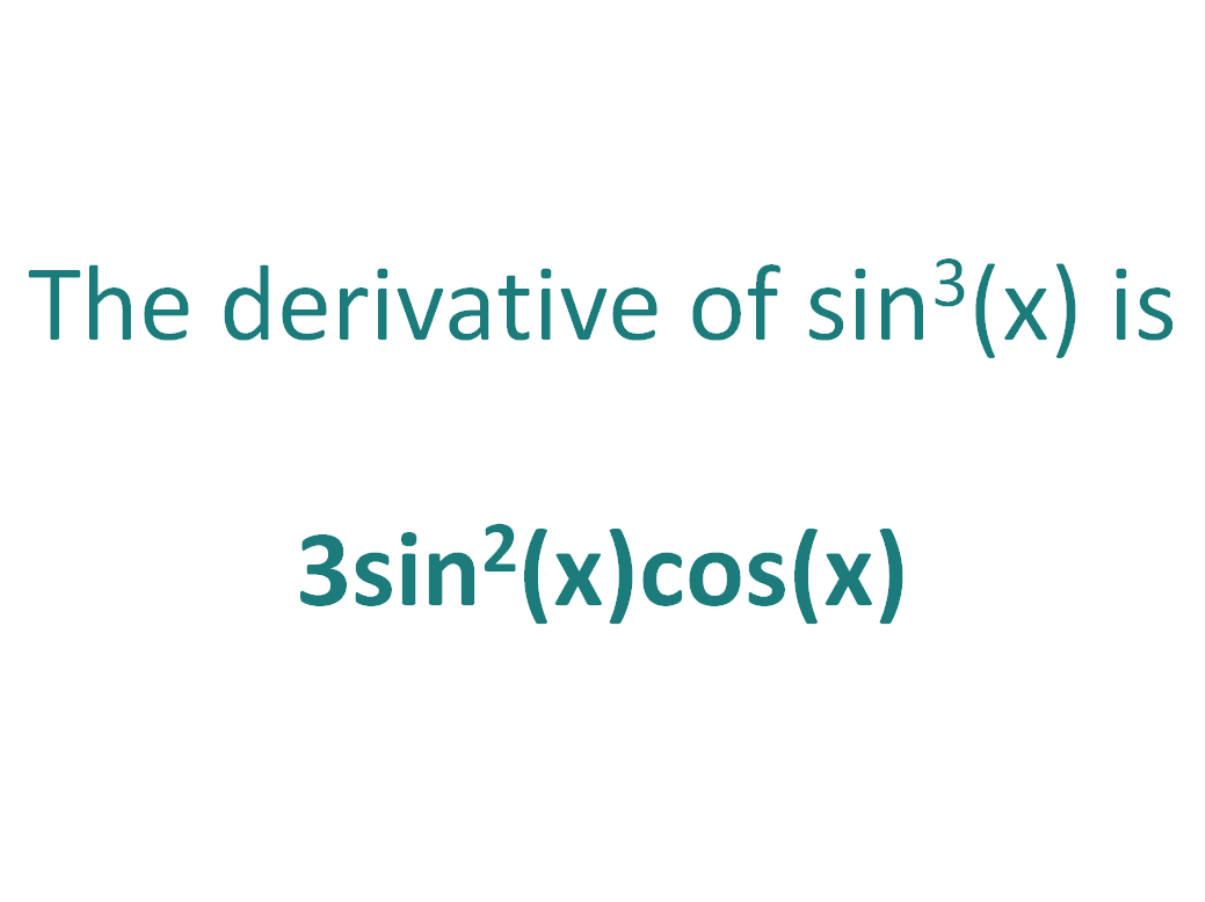# The Derivative of sin^3(x)The derivative of sin^3(x) is 3sin^2(x)cos(x)

## How to calculate the derivative of sin^3x

Note that in this post we will be looking at differentiating sin3(x) which is not the same as differentiating sin(3x). Here is our post dealing with how to differentiate sin(3x).

The chain rule is useful for finding the derivative of a function which could have been differentiated had it been in x, but it is in the form of another expression which could also be differentiated if it stood on its own.

In this case:

• We know how to differentiate sin(x) (the answer is cos(x))
• We know how to differentiate x3 (the answer is 3x2)

This means the chain rule will allow us to perform the differentiation of the expression sin^3(x).

#### Using the chain rule to find the derivative of sin^3(x)

Although the expression sin3x contains no parenthesis, we can still view it as a composite function (a function of a function).

We can write sin3x as (sin(x))3.

Now the function is in the form of x3, except it does not have x as the base, instead it has another function of x (sin(x)) as the base.

Let’s call the function of the base g(x), which means:

g(x) = sin(x)

From this it follows that:

sin(x)3 = g(x)3

So if the function f(x) = x3 and the function g(x) = sin(x), then the function (sin(x))3 can be written as a composite function.

f(x) = x3

f(g(x)) = g(x)3 (but g(x) = sin(x))

f(g(x)) = (sin(x))3

Let’s define this composite function as F(x):

F(x) = f(g(x)) = (sin(x))3

We can find the derivative of sin^3x (F'(x)) by making use of the chain rule.

The Chain Rule:
For two differentiable functions f(x) and g(x)

If F(x) = f(g(x))

Then the derivative of F(x) is F'(x) = f’(g(x)).g’(x)

Now we can just plug f(x) and g(x) into the chain rule.

How to find the derivative of sin^3(x) using the Chain Rule:

Using the chain rule, the derivative of sin^3(x) is 3sin2(x)cos(x)

Finally, just a note on syntax and notation: sin^3(x) is sometimes written in the forms below (with the derivative as per the calculations above). Just be aware that not all of the forms below are mathematically correct.

## The Second Derivative Of sin^3(x)

To calculate the second derivative of a function, you just differentiate the first derivative.

From above, we found that the first derivative of sin^3x = 3sin2(x)cos(x). So to find the second derivative of sin^2x, we just need to differentiate 3sin2(x)cos(x).

We can use the product rule and trig identities to find the derivative of 3sin2(x)cos(x). We get an answer of -3sin3x + 3cos(x)sin(2x)

The second derivative of sin^3(x) is -3sin3x + 3cos(x)sin(2x)